# GEOMETRICAL CONSTRUCTION – LINES

Welcome to Class !!

In today’s Basic Technology class, We will be discussing Geometrical Construction of Lines. We hope you enjoy the class!## Bisecting lines

When we construct or draw geometric figures, we often need to bisect lines or angles. Bisect means to cut something into two equal parts. There are different ways to bisect a line segment.

### Bisecting a line segment with a ruler

1. Read through the following steps.
• Step 1: Draw line segment AB and determine its midpoint.• Step 2: Draw any line segment through the midpoint.

The small marks on AF and FB show that AF and FB are equal.CD is called a bisector because it bisects AB. AF = FB.

Use a ruler to draw and bisect the following line segments: AB = 6 cm and XY = 7 cm.

In Grade 6, you learnt how to use a compass to draw circles, and parts of circles called arcs. We can use arcs to bisect a line segment.

### Bisecting a line segment with a compass and ruler

1. Read through the following steps.
• Step 1

Place the compass on one endpoint of the line segment (point A). Draw an arc above and below the line. (Notice that all the points on the arc above and below the line are the same distance from point A.)• Step 2

Without changing the compass width, place the compass on point B. Draw an arc above and below the line so that the arcs cross the first two. (The two points where the arcs cross are the same distance away from point A and from point B.)• Step 3

Use a ruler to join the points where the arcs intersect. This line segment (CD) is the bisector of AB.Intersect means to cross or meet.

A Perpendicular line is a line that meets another line at an angle of 90°.

Notice that CD is also perpendicular to AB. So it is also called a perpendicular bisector.

Work in your exercise book. Use a compass and a ruler to practise drawing perpendicular bisectors on line segments.

Try this!

Work in your exercise book. Use only a protractor and ruler to draw a perpendicular bisector on a line segment. (Remember that we use a protractor to measure angles.)

## Constructing perpendicular lines

### A perpendicular line from a given point

1. Read through the following steps.
• Step 1

Place your compass on the given point (point P). Draw an arc across the line on each side of the given point. Do not adjust the compass width when drawing the second arc.• Step 2

From each arc on the line, draw another arc on the opposite side of the line from the given point (P). The two new arcs will intersect.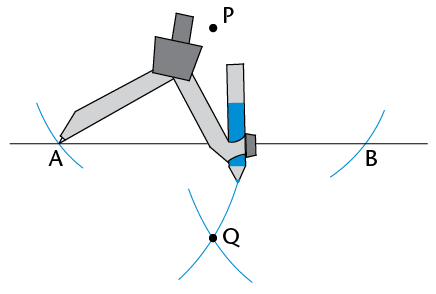• Step 3

Use your ruler to join the given point (P) to the point where the arcs intersect (Q).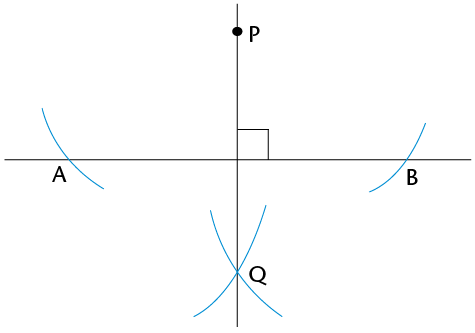PQ is perpendicular to AB. We also write it like this: PQ âŠ¥ AB.

1. Use your compass and ruler to draw a perpendicular line from each given point to the line segment:### A perpendicular line at a given point on a line

1. Read through the following steps.
• Step 1

Place your compass on the given point (P). Draw an arc across the line on each side of the given point. Do not adjust the compass width when drawing the second arc.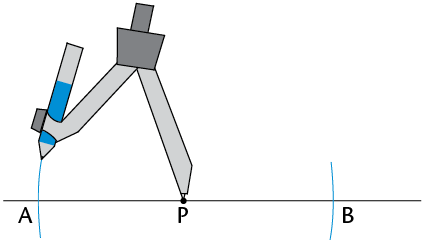• Step 2

Open your compass so that it is wider than the distance from one of the arcs to the point P. Place the compass on each arc and draw an arc above or below the point P. The two new arcs will intersect.• Step 3

Use your ruler to join the given point (P) and the point where the arcs intersect (Q).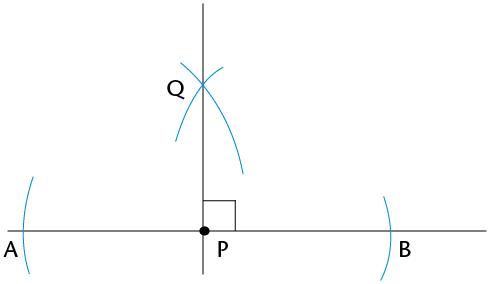PQ âŠ¥ AB

1. Use your compass and ruler to draw a perpendicular at the given point on each line: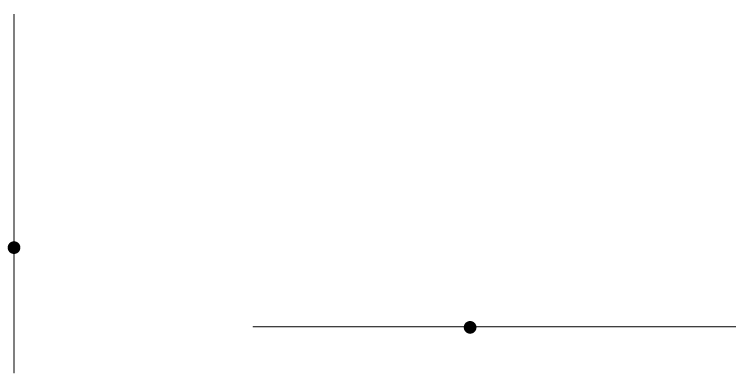### Bisecting angles without a protractor

1. Read through the following steps.
• Step 1

Place the compass on the vertex of the angle (point B). Draw an arc across each arm of the angle.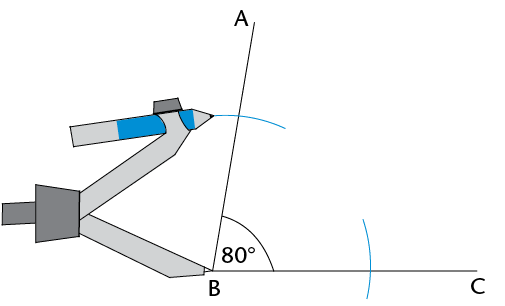• Step 2

Place the compass on the point where one arc crosses an arm and draw an arc inside the angle. Without changing the compass width, repeat for the other arm so that the two arcs cross.• Step 3

Use a ruler to join the vertex to the point where the arcs intersect (D).DB is the bisector of $$\hat{ABC}$$.

1. Use your compass and ruler to bisect the angles below.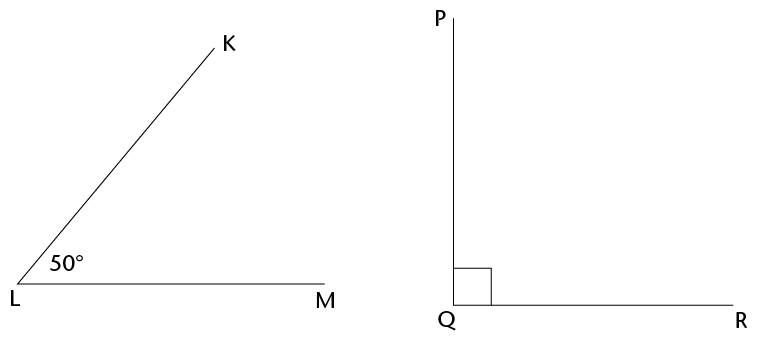We have come to the end of this class. We do hope you enjoyed the class?

Should you have any further question, feel free to ask in the comment section below and trust us to respond as soon as possible.

In our next class, we will be talking about Angles. We are very much eager to meet you there.

Are you a Parent? Share your quick opinion and win free 2-month Premium Subscription

Don`t copy text!